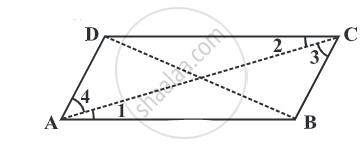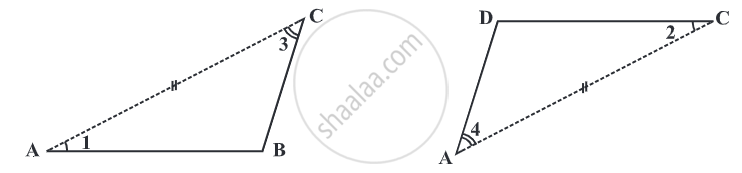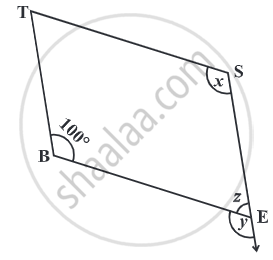The opposite angles of a parallelogram are of equal measure.

Given: ABCD is a parallelogram.To Prove: m∠ A = m∠C

Construction: Draw diagonal AC and diagonal BD.

To Prove:

AC and BD are the diagonals of the parallelogram,

∠ BAC = ∠ ACD and ∠ DAC = ∠ ACB......(pair of alternate angles) and bar(AC) is common side,

∠ BAC = ∠ ACD    ......(pair of alternate interior angles)

∠ DAC = ∠ ACB    ......(pair of alternate interior angles)

Side AC = Side AC ......(common side)

∆ABC ≅ ∆CDA       ......(ASA congruency criterion)This shows that ∠B and ∠D have same measure.  In the same way you can get, m∠A = m ∠C.

Alternatively, ∠ BAC = ∠ ACD and ∠ DAC = ∠ ACB

We have, m∠ A = ∠ BAC + ∠ DAC and ∠ ACB + ∠ ACD = m∠C.

m∠A = m ∠C.

Hence Proved.

Example

In the given Fig, BEST is a parallelogram. Find the values x, y, and z.S is opposite to B.
So,
x = 100°  ........(opposite angles property)
y = 100° ..........(a measure of angle corresponding to ∠ x)
z = 80°   ..........(since ∠y, ∠z is a linear pair)
If you would like to contribute notes or other learning material, please submit them using the button below.

Shaalaa.com

To Prove that the Opposite Angles of a Parallelogram are equal in measure [00:06:23]
S
0%